# Involute

## Definition

A curve that is obtained by attaching a string which is imaginary and then winding and unwinding it tautly on the curve given is called involute in differential geometry. Involute or evolvent is the locus of the free end of this string

The evolute of an involute of a curve is referred to that original curve. In other words, the locus of the center of curvature of a curve is called evolute and the traced curve itself is known as the involute of its evolute.

This is part of a special branch of geometry called differential Geometry of Curves. It talks about the smooth curves which lie in Euclidean space and the application of different methods of integral and differential calculus on them. The shapes related to some other curves are called involutes. This was discovered by Christine Huygens in 1673. He was a Dutch mathematician and a physicist.

## Involutes of the Curves

Let’s learn about the involutes of the different curves as shown below:

• Involute of a Circle
• Involute of a Catenary
• Involute of a Deltoid
• Involute of a Parabola
• Involute of an Ellipse

1) Involute of a Circle: It is similar to the Archimedes spiral.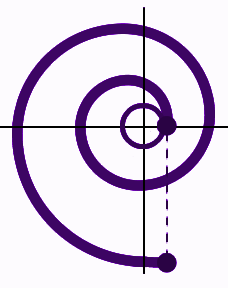2) Involute of a Catenary – It is a curve which is similar to hanging cable supported by its ends. So, it is a U shaped hanging chain which looks like a parabola. The tractrix is the involute of the catenary through the vertex.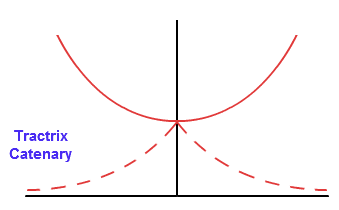3) Involute of a Deltoid- It is a tricuspid curve with three cusps. It resembles greek letter delta.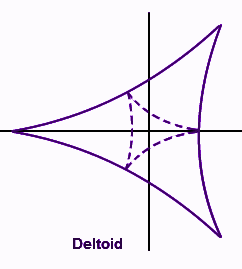4) Involute of a Parabola –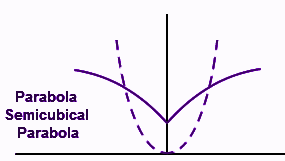5) Involute of an Ellipse –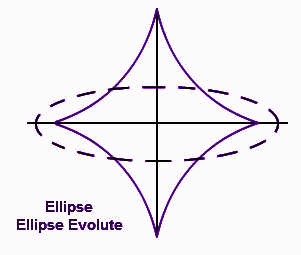## Equation

• Circle Involute
• Catenary Involute
• Deltoid Involute

Circle Involute: x = r (cos t + t sin t) , y = r (sin t – t cos t), where, r = radius of the circle, t = parameter of angle in radian.

Catenary Involute: x = t – tanh t, y = sech t, where t be the parameter.

Deltoid Involute: x = 2 r cos t + r cos 2t, y = 2 r sin t – r sin 2t

where, r = radius of rolling circle involved in formation of deltoid.

## Involute of a Circle

Involute of a circle is a practical concept, and also has various real life applications. It is mostly used in designing cogwheel or tooth-wheel which are used in rotating machines. It looks like an Archimedes spiral. Its parametric equations are shown below:

• In Cartesian Coordinates:

If r is the radius of the circle and the angle parameter is t, then

x = r (cos t + t sin t)

y = r (sin t – t cos t)

• In Polar Coordinates:

If r and θ are the parameters, then r = a sec α

θ = tan α – α, where, a be the radius of circle.

• Arc length of circle involute:

The length of the arc of the involute of the circle is

L = (r/2)t2

## How to Draw Involute

Let’s learn how to draw involute by following given steps:

1. Draw a few number of tangents to the points given on the curve
2. Pick 2 neighbouring tangent lines.
1. Extend these in opposite directions
2. Find their intersection point.
3. Now, Take that endpoint as center
4. Take the distance between the given center and the point of 1stt tangent.
5. An arc will be drawn.

As shown in the following figure, let L1 and L2 be two successive tangents

These intersection points are X and XA be the radius.

So, the arc AA1 is obtained.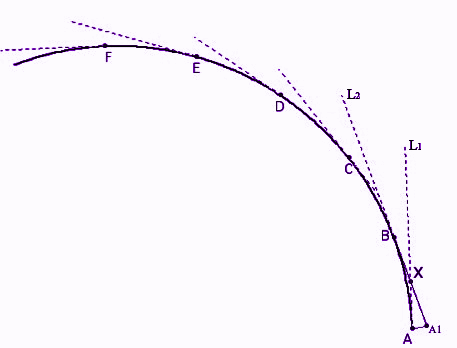1. Let us take another 2 neighbouring tangents L2 and L3.
1. Take their intersection point Y as center
2. Take distance YA1 as radius
3. Draw an arc A1A2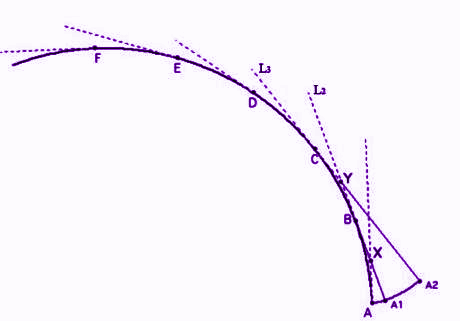1. Repeat the same process for the rest of the tangents. This way we will get a curve out of arcs constructed by now. And we will get the required involute of the curve.

## Application

The involutes of the curve have many applications in industries and businesses.

• Gear industries – To make teeth for two revolving machines and gears.
• Scroll compressing and Gas Compressing – These are made in this shape to reduce noise and to make them efficient.